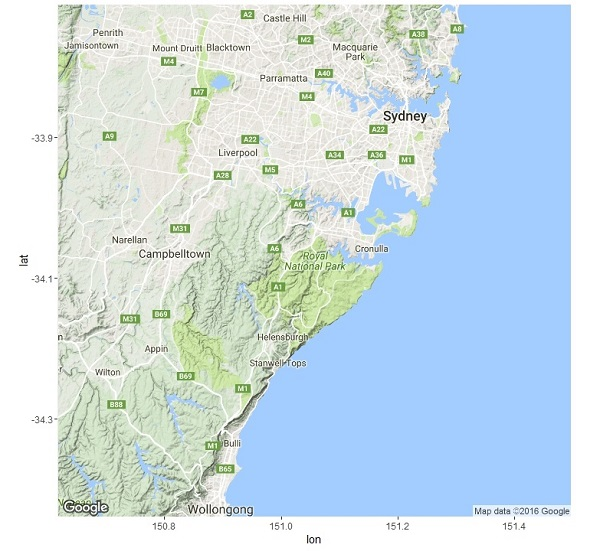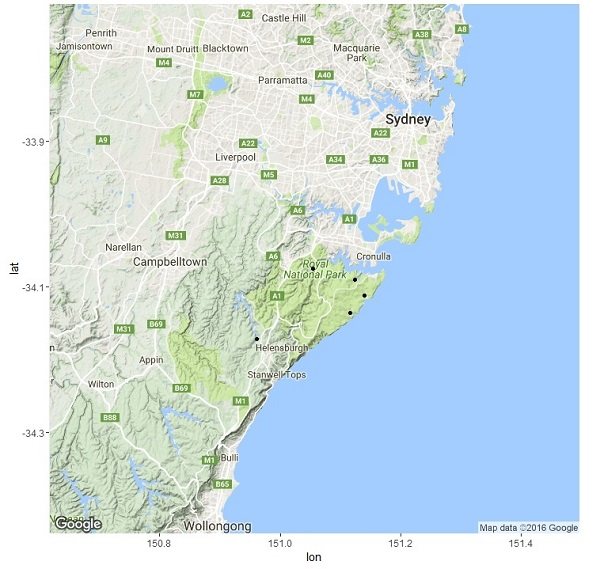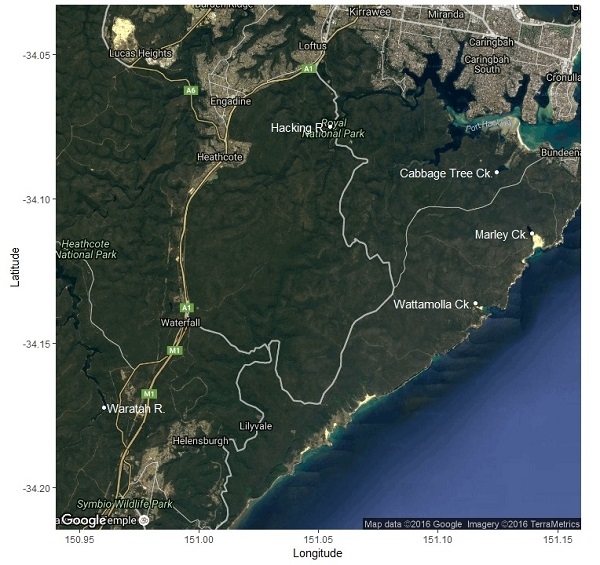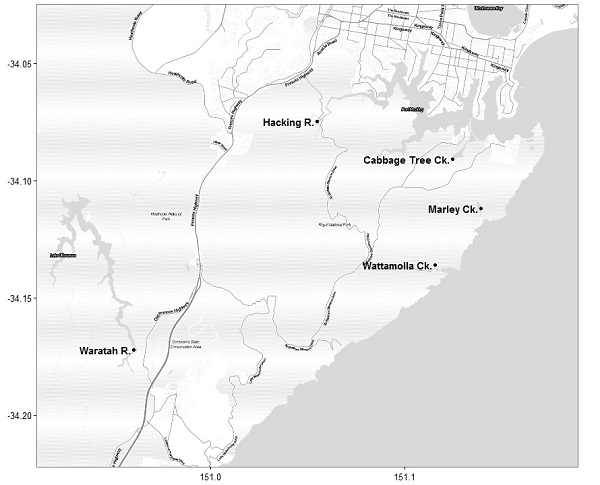Being able to make a simple map is a very useful skill for all sorts of applications. Here, we will introduce some of the basics of working with spatial data, and use R to produce simple and easy maps. You could use the same technique to make a map for a presentation, or a figure for a report or publication.

There are many packages that are useful for plotting and manipulating spatial data in R. For simplicity, we’re going to solely use the package ggmap for this exercise. To get started, install this package and load into R.

library(ggmap)

In this example, let’s say we collected data from five sites near Royal National Park in New South Wales, Australia, and want to display the locations we visited.

Using the function get_map, we can download an image from Google maps at any named location and then plot it with the function ggmap.

basemap <- get_map('Royal National Park')
ggmap(basemap)The argument of get_map is simply any place searchable on Google Maps (in this case ‘Royal National Park’) – easy!

To add point data for our five survey sites, we first need to make a new data frame holding the place name, latitude and longitude of each site (or import this data from a spreadsheet).

ptdata <- data.frame("PlaceName" = c(" Hacking R. ", " Cabbage Tree Ck. ",
" Marley Ck. ", " Wattamolla Ck. ",
" Waratah R. "),
"Long" = c(151.054823, 151.124566, 151.139216,
151.115645, 150.960431),
"Lat" = c(-34.074918, -34.090796, -34.111972,
-34.136046, -34.172308))                       

We can add these points to our map based on the Latitude (y) and Longitude (x) coordinates. It is important that the points and the other features on the map work on the same scale – or ‘projection’. These locations were estimated from Google Maps data for this reason – estimate your own via LatLong.net).

ggmap(basemap) + geom_point(data=ptdata, aes(x=Long, y=Lat))Well done -you made your first map with R! Easy! You may have noticed that ggmap uses familiar graphics code from the package ggplot (see plotting with ggplot).

By tweaking a few options, we can make this look more suitable for a presentation.

First, adjust some of the options in get_map:

• use bound to choose the extent/bounding box of the map. Here, we are using the minimum and maximum values of latitude and longitude from our site coordinates
• try maptype = "hybrid" to see the satellite imagery and roads data
• increase the zoom level to improve detail
bound <- c(left = min(ptdata$Long), bottom = min(ptdata$Lat),
right = max(ptdata$Long), top = max(ptdata$Lat))

Pres_basemap <- get_map(bound, zoom = 12, maptype = "hybrid")

Second, adjust some of the options for plotting:

geom_point tweaks for the dots at each site location:

• use colour = "white" to have the points stand out
• use size to change point size

geom_text tweaks for the labels for each site:

• use size to change text size
• use colour = "white" to have the labels stand out
• use hjust and vjust adjust the horizontal and vertical position of labels
• use labs to define the x and y axis labels
ggmap(Pres_basemap) +
geom_point(data = ptdata, aes(x=Long, y=Lat, label=PlaceName),
colour = "white") +
geom_text(data = ptdata,
aes(x=Long, y=Lat, label = PlaceName),
size = 4,
colour = "white",
hjust = "inward") +
labs(x = "Longitude", y = "Latitude")For a paper or report, you probably want a simpler black and white map.

• use bound to set the extent as above
• try get_stamenmap to get an austere basemap (eg. Stamen “toner-lite”)
• use theme_bw() from ggplot to get a stripped-back theme
Pub_bound <- c(left = min(ptdata$Long) - 0.05, bottom = min(ptdata$Lat) - 0.05,
right = max(ptdata$Long) + 0.05 , top = max(ptdata$Lat) + 0.05)
Pub_basemap <- get_stamenmap(Pub_bound, zoom = 13, maptype = "toner-lite")

ggmap(Pub_basemap) +
geom_point(data = ptdata, aes(x=Long, y=Lat, label=PlaceName)) +
geom_text(data = ptdata,
aes(x=Long, y=Lat, label = PlaceName),
size = 4,
fontface = "bold",
hjust = "right") +
theme_bw() +
theme(axis.title = element_blank())### Further help

Read the ggmap documentation for more examples and ideas.

ggmap cheat sheet from the National Centre for Ecological Analysis and Synthesis.

Author: Kingsley Griffin
Last updated:

##  "Wed Aug 03 09:00:33 2016"